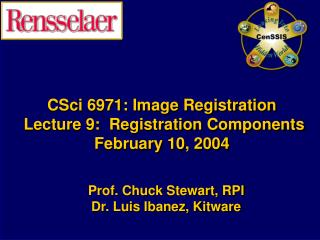DownloadDownload PresentationCSci 6971: Image Registration Lecture 9: Registration Components February 10, 2004

# CSci 6971: Image Registration Lecture 9: Registration Components February 10, 2004

Télécharger la présentation## CSci 6971: Image Registration Lecture 9: Registration Components February 10, 2004

- - - - - - - - - - - - - - - - - - - - - - - - - - - E N D - - - - - - - - - - - - - - - - - - - - - - - - - - -
##### Presentation Transcript

1. CSci 6971: Image Registration Lecture 9: Registration ComponentsFebruary 10, 2004 Prof. Chuck Stewart, RPI Dr. Luis Ibanez, Kitware

2. Registration Components Basic Registration Framework Lecture 9

3. Image Registration Framework FixedImage Metric MovingImage Interpolator Optimizer Transform Parameters Lecture 9

4. Other Image Metrics Mean Reciprocal Square Differences Lecture 9

5. 1 Match( A , B ) += ( 1 + λ∙Difference( index ) 2 ) Mean Reciprocal Squared Differences For each pixel in A Image A Image B Difference( index ) = A( index ) – B( index ) Lecture 9

6. j j i i Moving Image Grid Fixed Image Grid y’ y Space Transform x’ x Moving ImagePhysical Coordinates Fixed ImagePhysical Coordinates For each pixel in the Fixed Image Lecture 9

7. Mean Reciprocal Squared Differences #include "itkImage.h" #include "itkMeanReciprocalSquareDifferenceImageToImageMetric.h" #include "itkLinearInterpolateImageFunction.h" #include "itkTranslationTransform.h" typedef itk::Image< char, 2 > ImageType; ImageType::ConstPointerfixedImage = GetFixedImage(); ImageType::ConstPointermovingImage = GetMovingImage(); typedef itk::LinearInterpolateImageFunction<ImageType, double >InterpolatorType; InterpolatorType::Pointerinterpolator = InterpolatorType::New(); typedef itk::TranslationTransform< double, 2 > TransformType; TransformType::Pointertransform = TransformType::New(); Lecture 9

8. Mean Reciprocal Squared Differences typedef itk::MeanReciprocalSquareDifferenceImageToImageMetric< ImageType,ImageType > MetricType; MetricType::Pointermetric = MetricType::New(); metric->SetInterpolator( interpolator ); metric->SetTransform( transform ); metric->SetFixedImage( fixedImage ); metric->SetMovingImage( movingImage ); MetricType::TransformParametersTypetranslation( Dimension ); translation = 12; translation = 27; double value = metric->GetValue( translation ); Lecture 9

9. Evaluating many matches y y Transform x x Fixed Image Moving Image Lecture 9

10. Plotting the Metric Mean Reciprocal Squared Differences Transform Parametric Space Lecture 9

11. Plotting the Metric Mean Reciprocal Squared Differences Transform Parametric Space Lecture 9

12. Evaluating many matches y y Transform (-15,-25) mm x x Fixed Image Moving Image Lecture 9

13. Plotting the Metric Mean Reciprocal Squared Differences Transform Parametric Space Lecture 9

14. Plotting the Metric Mean Reciprocal Squared Differences Transform Parametric Space Lecture 9

15. Watch over your optimizer Example:Optimizer registering an image with itself starting at (-15mm, -25mm) Lecture 9

16. Plotting the Optimizer’s Path Mean Reciprocal Squared Differences Step Length = 1.0 mm Lecture 9

17. Plotting the Optimizer’s Path Mean Reciprocal Squared Differences Step Length = 2.0 mm Lecture 9

18. Plotting the Optimizer’s Path Mean Reciprocal Squared Differences Step Length = 5.0 mm Lecture 9

19. Plotting the Optimizer’s Path Mean Reciprocal Squared Differences Step Length = 10.0 mm Lecture 9

20. Plotting the Optimizer’s Path Mean Reciprocal Squared Differences Step Length = 20.0 mm Lecture 9

21. Plotting the Optimizer’s Path Mean Reciprocal Squared Differences Step Length = 40.0 mm Lecture 9

22. Quiz #1 If the Metric is NoisyWhere is the noise coming from ? Lecture 9

23. Smoothing the Image Lecture 9

24. Evaluating many matches y y Transform x x Fixed Image Moving Image Lecture 9

25. Plotting the Smoothed Metric Mean Reciprocal Squared Differences Transform Parametric Space Lecture 9

26. Plotting the Smoothed Metric Mean Reciprocal Squared Differences Transform Parametric Space Lecture 9

27. Watch over your optimizer Example:Optimizer registering an image with itself starting at (-15mm, -25mm) Lecture 9

28. Plotting the Optimizer’s Path Mean Reciprocal Squared Differences Step Length = 1.0 mm Lecture 9

29. Plotting the Optimizer’s Path Mean Reciprocal Squared Differences Step Length = 2.0 mm Lecture 9

30. Plotting the Optimizer’s Path Mean Reciprocal Squared Differences Step Length = 5.0 mm Lecture 9

31. Plotting the Optimizer’s Path Mean Reciprocal Squared Differences Step Length = 10.0 mm Lecture 9

32. Plotting the Optimizer’s Path Mean Reciprocal Squared Differences Step Length = 20.0 mm Lecture 9

33. Plotting the Optimizer’s Path Mean Reciprocal Squared Differences Step Length = 40.0 mm Lecture 9

34. Evaluating many matches y y Transform (-15,-25) mm x x Fixed Image Moving Image Lecture 9

35. Plotting the Smoothed Metric Mean Reciprocal Squared Differences Transform Parametric Space Lecture 9

36. Plotting the Smoothed Metric Mean Reciprocal Squared Differences Transform Parametric Space Lecture 9

37. Watch over your optimizer Example:Optimizer registering an image shifted by (-15mm, -25mm)The optimizer starts at (0mm,0mm) Lecture 9

38. Plotting the Optimizer’s Path Mean Reciprocal Squared Differences Step Length = 1.0 mm Lecture 9

39. Plotting the Optimizer’s Path Mean Reciprocal Squared Differences Step Length = 2.0 mm Lecture 9

40. Plotting the Optimizer’s Path Mean Reciprocal Squared Differences Step Length = 5.0 mm Lecture 9

41. Plotting the Optimizer’s Path Mean Reciprocal Squared Differences Step Length = 10.0 mm Lecture 9

42. Plotting the Optimizer’s Path Mean Reciprocal Squared Differences Step Length = 20.0 mm Lecture 9

43. Plotting the Optimizer’s Path Mean Reciprocal Squared Differences Step Length = 40.0 mm Lecture 9

44. Other Image Metrics Multi – ModalityRegistration Lecture 9

45. Multiple Image Modalities Number of pairs Lecture 9

46. Multiple Image Modalities More possible pairs Lecture 9

47. Intuitive Notion of Joint Entropy The More Pairs ExistThe Larger the Joint Entropy Lecture 9

48. Mutual Information Reduction of Number of PairsReduction of Joint Entropy Lecture 9

49. Mutual Information Mutual Information =Joint Entropy ( Image A, Image B )- Entropy Image A- Entropy Image B Lecture 9

50. Mattes Mutual Information #include "itkImage.h" #include "itkMattesMutualInformationImageToImageMetric.h" #include "itkLinearInterpolateImageFunction.h" #include "itkTranslationTransform.h" typedef itk::Image< char, 2 > ImageType; ImageType::ConstPointerfixedImage = GetFixedImage(); ImageType::ConstPointermovingImage = GetMovingImage(); typedef itk::LinearInterpolateImageFunction<ImageType, double >InterpolatorType; InterpolatorType::Pointerinterpolator = InterpolatorType::New(); typedef itk::TranslationTransform< double, 2 > TransformType; TransformType::Pointertransform = TransformType::New(); Lecture 9